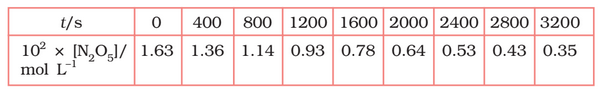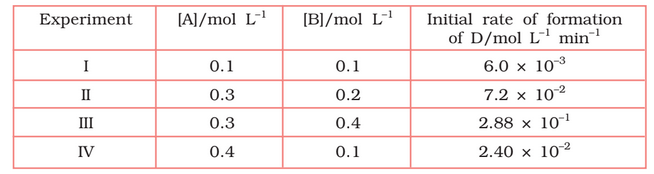## Filters

Sort by :
Clear All
Q

4.15 (5)   The experimental data for decomposition of  in gas phase at 318K are given below:Calculate the rate constant.

From the log graph, the slope of the graph is =                                            = -k/2.303                             ..(from log equation)        On comparing both the equation we get,

4.15 (4)    The experimental data for decomposition of  in gas phase at 318K are given below:What is the rate law ?

Here, the reaction is in first order reaction because its log graph is linear. Thus rate law can be expessed  as

4.15 (3)   The experimental data for decomposition of in gas phase at 318K are given below:Draw a graph between   and t.

0 1.63 -1.79 400 1.36 -1.87 800 1.14 -1.94 1200 0.93 -2.03 1600 0.78 -2.11 2000 0.64 -2.19 2400 0.53 -2.28 2800 0.43 -2.37 3200 0.35 -2.46

4.15 (2)   The experimental data for decomposition of   in gas phase at 318K are given below:Find the half-life period for the reaction.

Half life of the reaction is-
time corresponding to the mol/ L  = 81.5 mol /L is the half life of the reaction.  From the graph, the answer shoul be in the range of 1400 s to 1500 s.

4.15 (1)    The experimental data for decomposition of

in gas phase at 318K are given below:Plot against t.

On increasing time, the concentration of  gradually decreasing exponentially.

4.14   The half-life for radioactive decay of is 5730 years. An archaeological artifact containing wood had only 80% of the 14C found in a living tree. Estimate the age of the sample.

Given , half-life of radioactive decay = 5730 years So,                  per year we know that, for first-order reaction,                  = 1845 years (approximately) Thus, the age of the sample is 1845 years

4.13 (3)   Calculate the half-life of a first order reaction from their rate constants given  below:

The half-life for the first-order reaction is                                                                               = 0.693/4                                                                             =  0.173 year (approximately)

4.13 (2)   Calculate the half-life of a first order reaction from their rate constants given below:

the half-life for the first-order reaction is expressed as ;           =  0.693/2           = 0.35 min (approx)

4.13 (1)   Calculate the half-life of a first order reaction from their rate constants given below:

We know that, half-life () for first-order reaction =                                                             =

4.12   The reaction between A and B is first order with respect to A and zero order
with respect to B. Fill in the blanks in the following table:The given reaction is first order wrt A and zero order in wrt B. So, the rate of reaction can be expressed as;   Rate = k[A] from exp 1, k = 0.2 per min. from experiment 2nd, [A] =  from experiment 3rd,             from the experiment 4th, from here [A] = 0.1 mol/L

4.11   The following results have been obtained during the kinetic studies of the reaction:
2A + B  C + DDetermine the rate law and the rate constant for the reaction.

Let assume the rate of reaction wrt A is  and wrt B is  . So, the rate of reaction is expressed as- Rate =  According to given data,  these are the equation 1, 2, 3 and 4 respectively Now,  divide the equation(iv) by (i) we get, from here we calculate that  Again, divide equation (iii) by (ii) from here we can calculate the value of y is 2 Thus, the rate law is now,   So,         ...

4.10   In a reaction between A and B, the initial rate of reaction (r0) was measure for different initial concentrations of A and B as given below:What is the order of the reaction with respect to A and B?

we know that  rate law () =  As per data   these are the equation 1, 2 and 3 respectively Now, divide eq.1 by equation2, we get from here we calculate that y = 0 Again, divide eq. 2 by Eq. 3, we get Since y =0 also substitute the value of y  So,   =  =  taking log both side we get,     = 1.496     = approx 1.5 Hence the order of reaction w.r.t A is 1.5 and w.r.t B is 0(zero)

1.26     Explain the following with suitable examples:

(v)12-16 and 13-15 group compounds

(v)12-16 and 13-15 group compounds 12-16 group compounds: Compounds formed between elements of group 12 and elements of group 16 are called 12-16 group compounds. Example: ZnS 13-15 group compounds: Compounds formed between elements of group 13 and elements of group 15 are called 13-15 group compounds. Example: GaAs

1.26     Explain the following with suitable examples:

(iv) Antiferromagnetism

(iv) Antiferromagnetism :  Substances like MnO showing antiferromagnetism have domain structure similar to ferromagnetic substance, but their domains are oppositely oriented and cancel out each other's magnetic moment as shown in the figure.

1.26    Explain the following with suitable examples:

(iii)    Ferrimagnetism

(iii)    Ferrimagnetism :  When the magnetic moments of the domains in the substance are aligned in parallel and anti-parallel directions in unequal numbers than ferrimagnetism is observed. Refer to the given figure. They are weakly attracted by a magnetic field as compared to ferromagnetic substances. Example:. These substances also lose ferrimagnetism on heating and become paramagnetic.

1.26     Explain the following with suitable examples:

(ii) Paramagnetism

(ii) Paramagnetism :  The substances that are weakly attracted by a magnetic field are called paramagnetic substances. They are magnetised in a magnetic field in the same direction. They lose their magnetism in the absence of a magnetic field. Paramagnetism is due to the presence of one or more unpaired electrons which are attracted by the magnetic field. Example:.

1.26     Explain the following with suitable examples:

(i) Ferromagnetism

(i) Ferromagnetism Substances that are attracted very strongly by a magnetic field are called ferromagnetic substances. Example: iron, cobalt, nickel, gadolinium and CrO2. Besides strong attractions, these substances can be permanently magnetised. The metal ions of ferromagnetic substances are grouped together into small regions called domains. Thus, each domain acts as a tiny magnet. In an...

1.25     If NaCl is doped with 10–3 mol % of SrCl2, what is the concentration of cation vacancies?

NaCl is doped with 10–3 mol % of  Concentration in % so that take a total of 100 mol of solution. Moles of NaCl = 100 - moles of  Moles of  is very less , so we can neglect them. Moles of NaCl =100 1 mole of NaCl is dipped with =   mol of . So cation vacanties per mole of NaCl = mol   particles  So cation vacancies per mol of NaCl =                                                         ...

1.25     Aluminium crystallises in a cubic close-packed structure. Its metallic radius is 125 pm.
(ii) How many unit cells are there in 1.00 cm3 of aluminium?

For cubic close-packed structure, we have  Given :                          the volume of one unit cell                                                                                    Total unit cells in 1.00 cm3

1.24 Aluminium crystallises in a cubic close-packed structure. Its metallic radius is 125 pm.
(i) What is the length of the side of the unit cell?

For  cubic close-packed structure, we have  Given :
Exams
Articles
Questions